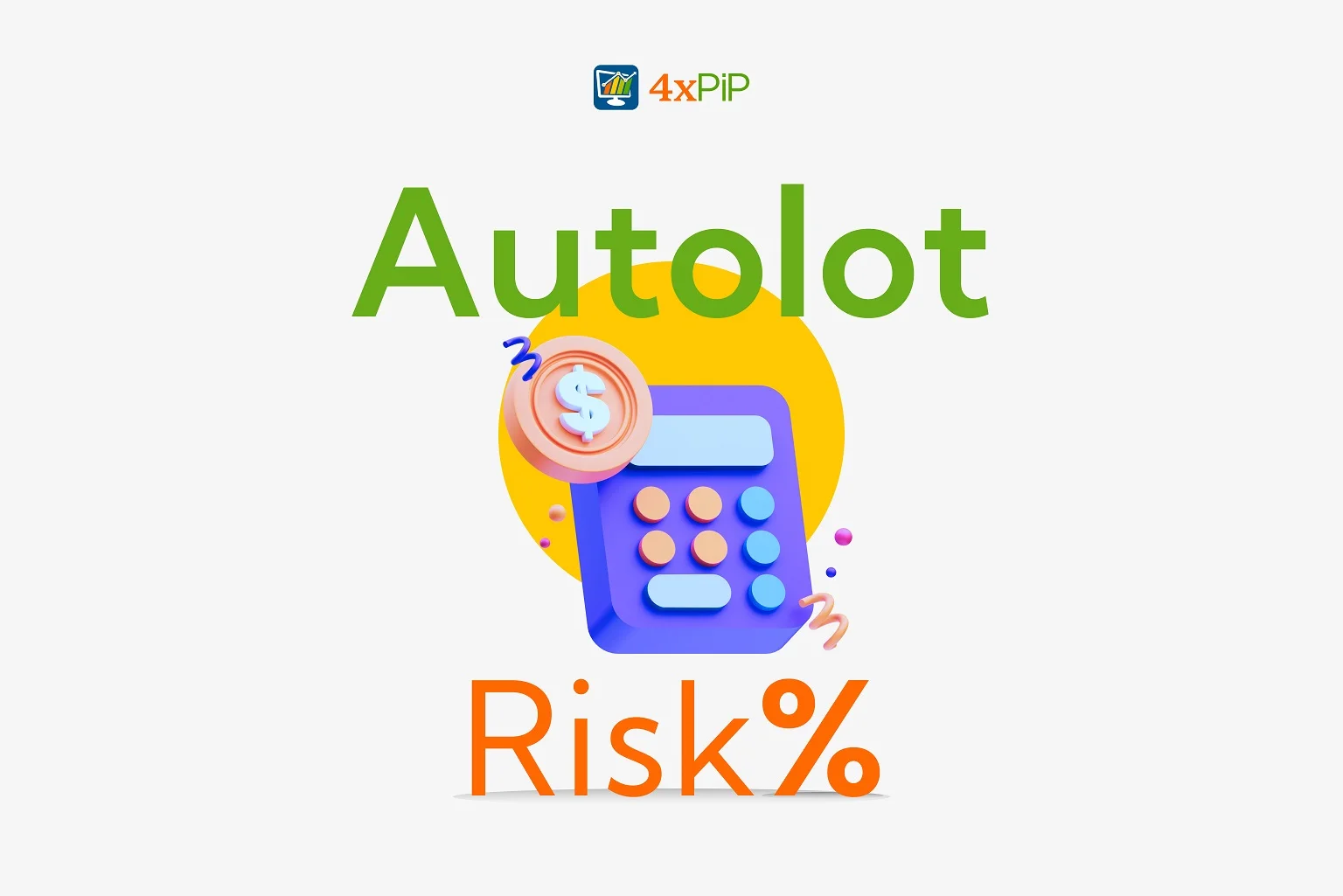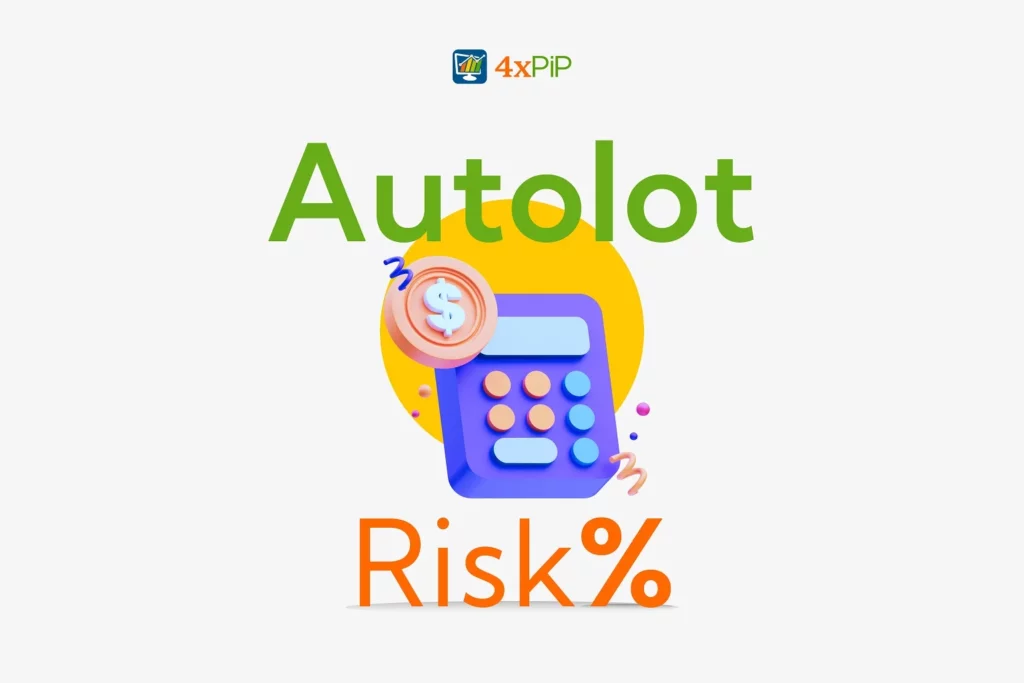# What are lots in Forex?What are lots in Forex? In forex trading, 1 lot equals 100,000 units of the base currency. For example, if an investor enters a long 1 lot EUR/USD trade, they are buying 100,000 units of the Euro (the base currency) against the USD (the counter currency).

## How to Calculate Auto Lot Size?

The ideal position size can be calculated using the following formula:

`LotSize = Account Equity x Risk You are taking / (Stoploss in pips x Pip Value)`

Consider a scenario where a forex trader has a starting account equity of \$10,000 and aims to execute a trade with a risk per trade of 2% with a Stoploss of 67 pips. Applying the above formula yields:

Lot size = Account Equity x Risk you are taking / (Stoploss in pips x Pip Value)

Lot size = \$10,000 x 0.02 / (67 pips x \$10)

Lot size = \$200 / (67 pips x \$10)

Lot size = \$0.29

Most commonly, lot size is rounded off to 2 decimal places.

If this USD 10,000 account, risking 2% (USD 200) and makes 10% in profit on a 10 Risk:Reward trade, you’ll now have an account balance of USD 12,000. Now on the next trade, your risk of 2% per trade will be USD 240. By using risk-based position sizing you will naturally scale your risk up and down depending on the size of your account and take advantage of the compounding gains effect.